Student Resources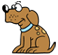Don't want

Big Ideas Math: Red

Chapter 1: Operations with Integers (pp. 1 - 1)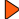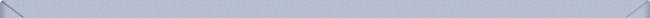Math Tool Paper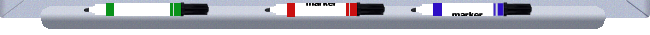Chapter 2: Rational Numbers and Equations (pp. 48 - 95)Math Tool PaperChapter 3: Proportions and Variation (pp. 96 - 155)Math Tool PaperChapter 4: Percents (pp. 156 - 191)Math Tool PaperChapter 5: Similarity and Transformations (pp. 192 - 247)Math Tool PaperChapter 6: Surface Areas of Solids (pp. 248 - 295)Math Tool PaperChapter 7: Volumes of Solids (pp. 296 - 345)Math Tool PaperChapter 8: Data Analysis and Samples (pp. 346 - 381)Math Tool PaperChapter 9: Probability (pp. 382 - 419)Math Tool PaperAdditional Topics : (pp. 420 - 427)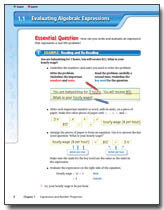Math Tool PaperAppendix A: My Big Ideas Projects (pp. A1 - A9)Support Links• Twenty Thousand Leagues Under the Sea: PDF   Audio
• A Journey to the Center of the Earth: PDF   Audio
• Around the World in Eighty Days: PDF   AudioAppendix B: Exploring Equations and Functions (Online Only)Math Tool Paper